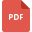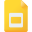# probability tutorialsProbability Introduction and ExamplesIntroduction to Probability with Sample Questions
Probability, Grade 5, United States, MathematicsProbability, Grade 6, United States, MathematicsExperimental Probability: Theory and Sample Problems
Probability, Grade 6, United States, MathematicsPractice Worksheet of Probability
Probability, Grade 4, United States, MathematicsProbability: Tree Diagrams
Tree Diagram, Grade 8, United States, ProbabilityTree diagram to find probability of two or more events
Probability, Grade 6, United States, Probability Theory, Tree DiagramProbability of Simple Events
Mathematics, Grade 8, United States, ProbabilityProbability: Case Study of Rolling a pair of dice
Probability, Grade 6, United States, MathematicsHow to find probability of some simple events?
Probability, Grade 6, United States, Probability TheoryA Brief Description of Experimental probability
Probability, Grade 8, United States, Mathematics